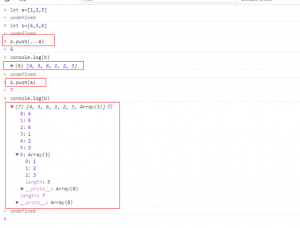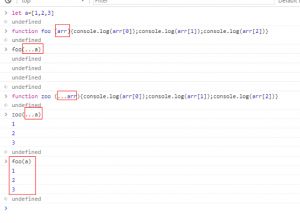1，模板字符串（推荐！）

2，块级作用域语法（推荐！）

3，扩展运算符（合并数组中的每项时，推荐使用！）

4，参数默认值(不是很推荐）

5，解构（不是很推荐）

6，对象字面量和属性的简洁表达式（不是很推荐）

7，动态属性名称（按实际需求，推荐使用）

8，箭头函数（推荐！）

9，for...of loops(for...of循环)  （按实际需求，推荐使用）

10，数字字面量 （按实际需求，推荐使用）

1，模板字符串

var fName = 'Peter', sName = 'Smith', age = 43, job= 'photographer';
var a = 'Hi, I\'m ' + fName + ' ' + sName + ', I\'m ' + age + ' and work as a ' + job + '.';
var b = `Hi, I'm \${ fName } \${ sName }, I'm \${ age } and work as a \${ job }.`;

2，块级作用域

JavaScript是使用函数作用域的，这就是为什么我们是为什么我们越来越频繁的使用匿名的立即执行函数表达式（iife）来实现整个JavaScript文件的封装。我们这么做是为了把所有的变量隔离在文件内从而避免变量冲突。

let declaration let命令

var a = 'car' ;
{
let a = 5;
console.log(a) // 5
}
console.log(a) // car

for (var i = 1; i < 5; i++){
setTimeout(() => { console.log(i); }, 1000);
}

{let i = 1; setTimeout(() => { console.log(i) }, 1000)}
{let i = 2; setTimeout(() => { console.log(i) }, 1000)}
{let i = 3; setTimeout(() => { console.log(i) }, 1000)}
{let i = 4; setTimeout(() => { console.log(i) }, 1000)}
{let i = 5; setTimeout(() => { console.log(i) }, 1000)}

varlet的另外一个区别是 let 不会像 var一样被变量提升

{
console.log(a); // undefined
console.log(b); // ReferenceError
var a = 'car';
let b = 5;
}

Const

const没有让变量完全不可变，只是锁定他的赋值，当你有一个复杂的变量（数组或者对象）的时候，值还是可以被修改的。

{
const d = [1, 2, 3, 4];
const dave = { name: 'David Jones', age: 32};
d.push(5);
dave.job = "salesman";
console.log(d);  // [1, 2, 3, 4, 5]
console.log(dave);  // { age: 32, job: "salesman", name: 'David Jones'}
}

Problem with block scoping functions函数块级作用域化带来的问题

{
bar(); // works
function bar() { /* do something */ }
}
bar();  // doesn't work

if ( something) {
function baz() { console.log('I passed') }
} else {
function baz() { console.log('I didn\'t pass') }
}
baz();

ES6之前，这两个函数声明都被变量提升，而且结果一定是I didn't pass 不论条件中的something是什么。但现在我们会得到输出ReferenceError, 因为 baz一直被限定在块级作用域内。

PS：该例子个人在chrome上实测，函数声明变量还是被提升了，依然会根据something来选择console内容。

3，扩展运算符

ES6介绍了...操作符，这个操作符指的就是‘扩展运算符‘。他的主要用途有两个：1. 将一个数组或者对象放到一个新的数组或者对象中 2. 将数组中的多个参数合并在一起

let a = [3, 4, 5];
let b = [1, 2, ...a, 6];
console.log(b);  // [1, 2, 3, 4, 5, 6]

function foo(a, b, c) {
console.log(`a=\${a}, b=\${b}, c=\${c}`)
}
let data = [5, 15, 2];
foo( ...data); // a=5, b=15, c=2

let car = { type: 'vehicle ', wheels: 4};
let fordGt = { make: 'Ford', ...car, model: 'GT'};
console.log(fordGt); // {make: 'Ford', model: 'GT', type: 'vehicle', wheels: 4}

let a = [1, 2, 3];
let b = [ ...a ];
let c = a;
b.push(4);
console.log(a);  // [1, 2, 3]
console.log(b);  // [1, 2, 3, 4] 不同的数组
c.push(5);
console.log(a);  // [1, 2, 3, 5]
console.log(c);  // [1, 2, 3, 5] 同一个数组

function foo(...args) {
console.log(args);
}
foo( 'car', 54, 'tree');  //  [ 'car', 54, 'tree' ]4，参数默认值

function foo( a = 5, b = 10) {
console.log( a + b);
}
foo();  // 15
foo( 7, 12 );  // 19
foo( undefined, 8 ); // 13
foo( 8 ); // 18
foo( null ); // 10 as null is coerced to 0

function foo( a ) { return a * 4; }
function bar( x = 2, y = x + 4, z = foo(x)) {
console.log([ x, y, z ]);
}
bar();  // [ 2, 6, 8 ]
bar( 1, 2, 3 ); //[ 1, 2, 3 ]
bar( 10, undefined, 3 );  // [ 10, 14, 3 ]

5，解构

let [ a, b, c ] = [ 6, 2, 9];
console.log(`a=\${a}, b=\${b}, c=\${c}`); //a=6, b=2, c=9

function foo() { return ['car', 'dog', 6 ]; }
let [ x, y, z ] = foo();
console.log(`x=\${x}, y=\${y}, z=\${z}`);  // x=car, y=dog, z=6

function bar() { return {a: 1, b: 2, c: 3}; }
let { a, c } = bar();
console.log(a); // 1
console.log(c); // 3
console.log(b); // undefined

function baz() {
return {
x: 'car',
y: 'London',
z: { name: 'John', age: 21}
};
}
let { x: vehicle, y: city, z: { name: driver } } = baz();
console.log(
`I'm going to \${city} with \${driver} in their \${vehicle}.`
); // I'm going to London with John in their car.

let { x: first, x: second } = { x: 4 };
console.log( first, second ); // 4, 4

a，首先是数组解构：

let [,b,]=[1,2,3]

let [a,..b]=[1,2,3]

b,对象的解构：

let {name,age,sex} = {name:'shotCat',age:18,sex:'man'}

6，对象字面量和属性的简洁表达法

let a = 4, b = 7;
let c = { a: a, b: b };
let concise = { a, b };
console.log(c, concise) // {a: 4, b: 7}, {a: 4, b: 7}

function foo() {
return {
name: 'Anna',
age: 56,
job: { company: 'Tesco', title: 'Manager' }
};
}
// pre ES6
let a = foo(), name = a.name, age = a.age, company = a.job.company;
// ES6 destructuring and concise parameters
let { name, age, job: {company}} = foo();

let person = {
name: 'Anna',
age: 56,
job: { company: 'Tesco', title: 'Manager' }
};
// method 1
function old1( person) {
var yearOfBirth = 2018 - person.age;
console.log( `\${ person.name } works at \${ person.job.company } and was born in \${ yearOfBirth }.`);
}
// method 2
function old1( person) {
var age = person.age,
yearOfBirth = 2018 - age,
name = person.name,
company = person.job.company;
console.log( `\${ name } works at \${ company } and was born in \${ yearOfBirth }.`);
}
// method 3
function es6({ age, name, job: {company}}) {
var yearOfBirth = 2018 - age;
console.log( `\${ name } works at \${ company } and was born in \${ yearOfBirth }.`);
}

7,动态属性名称

ES6添加了使用动态分配的键创建或添加属性的功能。

let  city= 'sheffield_';
let a = {
[ city + 'population' ]: 350000
};
a[ city + 'county' ] = 'South Yorkshire';
console.log(a); // {sheffield_population: 350000, sheffield_county: 'South Yorkshire' }

8,箭头函数

var foo = function( a, b ) {
return a * b;
}
let bar = ( a, b ) => a * b;

let arr = [ 5, 6, 7, 8, 'a' ];
let b = arr.map( item => item + 3 );
console.log(b); // [ 8, 9, 10, 11, 'a3' ]

var clickController = {
doSomething: function (..) {
var self = this;
'click',
function() { self.doSomething(..) },
False
);
}
};

let a = {
oneThing: ( a ) => {
let b = a * 2;
this.otherThing(b);
},
otherThing: ( b ) => {....}
};
a.oneThing(6);

9,for...of loops (for...of循环)

ES6新添加了一种方式来迭代数组中的每个值，这个方式是与已经存在的for...in的通过索引的循环方式不同。

let a = ['a', 'b', 'c', 'd' ];
// ES6
for ( var val of a ) {
console.log( val );
} // "a" "b" "c" "d"
// pre-ES6
for ( var idx in a ) {
console.log( idx );
}  // 0 1 2 3

for (var i = 0; i < array.length; i++) {
var obj = array[i];
}但是forEach当中是不能使用break和return。但使用for of就没有这个限制，并且更简洁。所以下次循环数组时，推荐使用for of！

10,Number Literals 数字字面量

ES5代码很好处理了十进制和十六进制的数字格式，但并未指定八进制的格式。实际上，八进制在严格模式中是被禁止使用的。

ES6 添加了一个全新的格式，在最开始的0后面添加一个o来声明一个八进制的数。与此同时，在es6中还添加了二进制格式。

Number( 29 )  // 29
Number( 035 ) // 35 in old octal form.
Number( 0o35 ) // 29 in new octal form
Number( 0x1d ) // 29 in hexadecimal
Number( 0b11101 ) // 29 in binary form